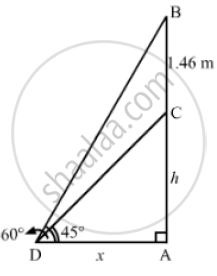# A Statue 1.46m Tall, Stands on the Top of a Pedestal. from a Point on the Ground, the Angle of Elevation of the Top of the Status is 60 and from the Same Point Find the Height of the Pedestal. - Mathematics

A statue 1.46m tall, stands on the top of a pedestal. From a point on the ground, the angle of elevation of the top of the status is 60 and from the same point, the angle of elevation of the top of the pedestal is 45 . Find the height of the pedestal.

#### Solution

Let AC be the pedestal and BC be the statue such that BC = 1.46m.
We have:

Let:
AC = hm and AD = xm(AC)/(AD) = tan 45° = 1
⇒ h/x = 1
⇒ h = x
Or,
x = h

Now, in the right ΔADB,we have:
(AB)/(AD) = tan 60° = sqrt(3)
(h+1.46)/x = sqrt(3)
On putting x  = h in the above equation, we get

(h+1.46)/h = sqrt(3)

⇒ h +  1.46 = sqrt(3)h

⇒ h( sqrt(3)-1) = 1.46

⇒ h = 1.46/( sqrt(3)-1) = 1.46/0.73 = 2m

Hence, the height of the pedestal is 2 m.

Concept: Heights and Distances
Is there an error in this question or solution?
Chapter 14: Height and Distance - Exercises

#### APPEARS IN

RS Aggarwal Secondary School Class 10 Maths
Chapter 14 Height and Distance
Exercises | Q 8

Share